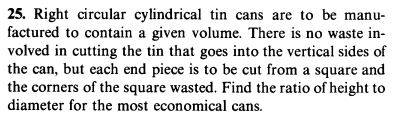# Minimum/Maximum problem

Karol

## Homework Statement## Homework Equations

Maxima/minima are where the first derivative is 0

## The Attempt at a Solution

Surface area of the whole metal for creating the can (the end pieces i take as squares):
$$A=2\pi h+8r^2,~~V=\pi r^2 h~~\rightarrow~h=\frac{V}{\pi r^2}$$
$$A=2\pi \frac{V}{\pi r^2}+8r^2,~~A'=2V(-2)r^{-3}+16r=16r-\frac{4V}{r^3}$$
$$A'=0~\rightarrow~4r^4=V$$
I can't get rid of the volume V

#### Attachments

Staff Emeritus
Homework Helper
Gold Member
You need to think more about your expression for the area contribution from the mantle area. It has the wrong physical dimension.

Karol
$$A=2\pi r h+8r^2=2\pi r \frac{V}{\pi r^2}+8r^2=2\frac{V}{r}+8r^2$$
$$A'=-2Vr^{-2}+16r,~~A'=0~\rightarrow~8r^3=V$$
No good

Staff Emeritus
Homework Helper
Gold Member
Note that you are looking for the ratio ##\xi =h/2r## that minimises the area for a fixed volume.

Karol
$$\frac{h}{2r}=\frac{\frac{V}{\pi r^2}}{\sqrt{V}}$$
Can't get rid of V

Staff Emeritus
Homework Helper
Gold Member
Yes you can. You are not using the information that you have regarding how V is expressed in terms of the ratio.

•QuantumQuest
Karol
##V=2\pi r^2h~## is the only relation regarding V, so i can't express V in terms of the ratio ##~\frac{h}{2r}##
besides, i used already ##~V=2\pi r^2h~## in ##~A=2\pi r h+8r^2~##, so i can't use it again

Staff Emeritus
Homework Helper
Gold Member
##V=2\pi r^2h~## is the only relation regarding V, so i can't express V in terms of the ratio ##~\frac{h}{2r}##
besides, i used already ##~V=2\pi r^2h~## in ##~A=2\pi r h+8r^2~##, so i can't use it again
This is an assumption you make and that assumption is wrong. Why wouldn't you be able to use it again?

Karol
$$V=2\pi r^2h,~\xi=\frac{h}{2r},~~V=2\pi r^3\xi$$
$$\xi=\frac{V^{2/3}}{\pi r^2}=\frac{(2\pi r^3\xi)^{2/3}}{\pi r^2}=\frac{\sqrt{4}}{\pi^3}\xi^{2/3}$$
$$\Rightarrow~\xi^{1/3}=\frac{\sqrt{4}}{\pi^3}~~\rightarrow~\xi=\frac{4}{\pi^6}$$
$$V=2\pi r^2h,~\xi=\frac{h}{2r},~~V=4\pi r^3\xi$$
$$\xi=\frac{V^{2/3}}{\pi r^2}=\frac{(4\pi r^3\xi)^{2/3}}{\pi r^2}=\frac{\sqrt{4}}{\sqrt{\pi}}\xi^{2/3}$$
$$\Rightarrow~\xi^{1/3}=\frac{\sqrt{4}}{\sqrt{\pi}}~~\rightarrow~\xi=\frac{4}{\pi}$$